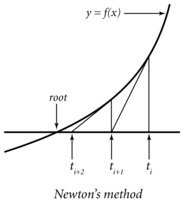Syllabus

Numerical analysis is the story of how functions, derivatives, integrals, and differential equations are handled as strings of numbers in the computer. At the heart of numerical analysis is an understanding of the speed of convergence of Taylor, Fourier, and other series expansions. Most scientists and engineers are sooner or later faced with computing tasks that require some knowledge of numerical analysis.

Topics:

• Series expansions: from calculus to computation
• Integrals as sums and derivatives as differences
• Interpolation, splines, and a second look at numerical calculus
• Numerical methods for ODE, initial-value problems
• Root finding, Newton's method, boundary-value problems
• Fourier transform, Fourier series, Shannon sampling theory
• Bandlimited interpolation, spectral methods
• Least-squares approximation
• Principal component analysis

The class will NOT cover partial differential equations (see 18.303), and will contain much less linear aglebra than 18.06.

Prerequisites: Calculus at the level of 18.01, 18.02, and 18.03. Some exposure to linear algebra (matrices) at the level of 18.06 helps, but is not required. The assignments will involve basic computer programming in the language of your choice (Matlab recommended; this class encourages you to learn Matlab if you don't already know it).

Reference material

The material will be inspired from various sources. Current version of the typeset notes: Feb 14 version, Feb 23 version, Mar 2 version, Mar 15 version, Mar 21 version, Apr 2 version, Apr 29 version, May 14 version, April 2014 version.

Useful books, not required:

• Burden and Faires, Numerical Analysis (more basic)
• Suli and Mayers, An Introduction to Numerical Analysis
• Stoer and Bulirsch, Introduction to Numerical Analysis (more advanced)
• Trefethen, Spectral methods in Matlab

Some code used in class:Who, when, and where

Date and Time: Tu-Th, 1:00-2:30, room 2-135. Instructor: Laurent Demanet. Office hours: W-F, 2:00-4:00, room 2-392.

Evaluation

50% homework, 20% in-class midterm, 30% in-class final.

The homework problem sets will consist of both theoretical problems and numerical experiments. No late copy will be allowed. The lowest score will be dropped. Collaboration is allowed, but the codes and copies you turn in must be original and written by you.

The midterm and final are open-book. No calculators, phones, or computers are allowed.

The midterm is scheduled on March 6 in class (1:00PM to 2:30PM in 2-135). The final is scheduled on May 24 from 9:00AM (sharp) to 12:00PM in Walker. Please arrive sufficiently ahead of schedule.